# Transitional densities of jump diffusion processes

## Moment equations for quadratic jump diffusions

DiffusionRjgqd is built around an algorithm that approximates solutions to the Kolmogorov forward equation (Hanson 2007): \begin{aligned} \frac{\partial}{\partial t} f(X_t|X_s) =& -\frac{\partial}{\partial X_t} [\mu(X_t,t) f(X_t|X_s)]+\frac{1}{2}\frac{\partial^2}{\partial X_t^2} [\sigma^2(X_t,t) f(X_t|X_s)]\\ &+\int\lambda(\nabla(X_t+J(X_t,\dot{z}_t)))f(\nabla(X_t+J(X_t,\dot{z}_t))|X_s)|\delta(\dot{z}_t)|d\phi(\dot{z}_t)d\dot{z}_t\\ &-\int\lambda(X_t)f(X_t|X_s)d\phi(\dot{z}_t)d\dot{z}_t, \end{aligned} where $$\phi(.)$$ gives the jump distribution and the elements $$\nabla(X_t+J(X_t,\dot{z}_t))$$ and $$|\delta(\dot{z}_t)|$$ have special meaning. Here, $$\nabla(X_t+J(X_t,\dot{z}_t))$$ has the effect of mapping the jumps $$J(X_t,\dot{z}_t)$$ in such a way that the state $$X_t$$ is reached as the result of a jump. For example, if $$J(X_t,\dot{z}_t) = \dot{z}_t$$ then $$\nabla(X_t+J(X_t,\dot{z}_t)) = X_t -\dot{z}_t$$. The function $$\nabla(.)$$ thus reverts the state of the process to that which it would have been prior to a jump occurance. The element $$|\delta(\dot{z}_t)|$$ on the other hand is simply the Jacobian corresponding to the function $$\nabla(.)$$. For example, if $$J(X_t,\dot{z}_t) = \dot{z}_t$$ and $$\nabla(X_t+J(X_t,\dot{z}_t)) = X_t -\dot{z}_t$$, then $$|\delta(\dot{z}_t)| =1$$. The approximation is based on calculating the conditional moment trajectories of a diffusion process over time and subsequently carrying these moments into a suitable surrogate density. The surrogate density is then used to approximate the solution to the Kolmogorov equation.

For example, given a scalar generalized quadratic diffusion:

$dX_t = (G_0(t)+G_1(t)X_t+G_2(t)X_t^2)dt +\sqrt{Q_0(t)+Q_1(t)X_t+Q_2(t)X_t^2}dB_t +dP_t,$ where $dP_t = J(X_t,\dot{z}_t)dN_t.$ for $$J(X_t,\dot{z}_t)$$ polynomial in $$X_t$$ where the intensity of the counting process $$N_t$$ is of the form $\lambda(X_t,\dot{z}_t) = \sum_{i=0}^{2}\lambda_i(t)X_t^i,$ it is possible to derive a system of differential equations that govern the evolution of the moments of the process over time.

Let $$m_i(t)$$ denote the $$i$$-th cumulant of $$X_t$$ given that the process assumes the initial value $$X_s$$. Then, for $$J(X_t,\dot{z}_t) = \dot{z}_t$$ (in DiffusionRjgqd, set argument Jtype = 'add' in for example JGQD.density() or JGQD.mcmc()):

\begin{aligned} \frac{\partial}{\partial t} m_i(t) &= i\bigg(\sum_{k=0}^{2}G_k(t)m_{i+k-1}(t)\bigg)\\ &+\frac{i(i-1)}{2}\bigg(\sum_{k=0}^{2}Q_k(t)m_{i+k-2}(t)\bigg)\mathbb{I}_{i\geq 2}\\ &+\lambda_0(t)\bigg(\sum_{k=0}^i {i\choose k}m_k(t) u_{i-k}-m_i(t) \bigg)\\ &+\sum_{l=1}^2\lambda_l(t)\bigg(\sum_{k=0}^i {i\choose k}m_{k+l-1}(t) u_{i-k+l}(t)-m_{i+l-1}(t) \bigg),\\ \end{aligned} subject to the initial conditions $$m_i(s) = X_s^i$$ for $$s<t$$ and $$i = 1,2,\ldots$$, where $$u_{i}$$ denotes the $$i$$-th non-central moment of the random variable $$\dot{z}_t$$. By solving these equations numerically via standard Runge-Kutta schemes and plugging the resulting values into a surrogate density such as a Normal distribution or a saddlepoint approximation, it is possible to accurately approximate the transitional density.

## An excess-factorization for the transitional density

Due to the dichotomous nature of jump diffusion processes a subtlety arises when attempting to approximate the transitional density by plugging its moments into a suitable surrogate density. On short transition horizons it is possible for the jump dynamics to create density characteristics that differ vastly from that of a purely diffuse process. For example, on a sufficiently short transition horizon, a purely diffuse process is approximately Normally distributed, whilst a jump diffusion (depending on the nature of the jump mechanism) may have fat tails with a very peaked distribution around the initial value of the process. As such, it does not always suffice to simply plug the moment trajectories of a jump diffusion into a surrogate density in order to approximate the transitional density. For these purposes we calculate the transitional density as a mixture density, factorizing the process in terms of its purely diffuse dynamics and an excess distribution, which accounts for the effect of the jump mechanism. That is, we calculate: $f_J(X_t|X_s) = P(N_t-N_s=0) f_{D}(X_t|X_s) +P(N_t-N_s>0)f_{E}(X_t|X_s)$

where $$f_J(X_t|X_s)$$ denotes the transitional density of the jump diffusion, $$f_D(X_t|X_s)$$ denotes the jump free counterpart to the jump diffusion and $$f_E(X_t|X_s)$$ denotes an excess distribution, and $$P(N_t-N_s=0)$$ is calculated as the solution to the equation:

$\frac{\partial}{\partial t} \log(P(N_t-N_s = 0)) = -\int \lambda(x,t)f_D(x|X_s)dx,$

In order to approximate the transitional density, the moments of $$f_J(X_t|X_s)$$ anf $$f_D(X_t|X_s)$$ are calculated after which one can deduce the moments of $$f_E(X_t|X_s)$$. Subsequently, the moments of $$f_D(X_t|X_s)$$ and $$f_E(X_t|X_s)$$ are used in conjunction with suitable surrogate densities (in DiffusionRjgqd we use the saddlepoint approximation) in order to approximate the transitional density.

# Non-linear jump diffusions

## The basic affine jump diffusion

An example of non-linear jump diffusion model often used in finance, termed the basic affine jump diffusion’’ (BAJD) (Eckner 2009) extends upon the popular CIR process by assuming that the paths of the process undergoes exponentially distributed jumps that occur with constant intensity. Thus, the corresponding SDE is given by the system:

\begin{aligned} dX_t &= a(b-X_t)dt +\sigma \sqrt{X_t}dB_t^{(1)}+dP_t\\ dP_t &= \dot{z}_tdN_t\\ \end{aligned} where the $$N_t$$ has a constant intensity, $$\lambda(X_t,t)= \lambda_0$$, and $$\dot{z}_t\sim \mbox{Exp}(\nu)$$.

Following the GQD framework for jump diffusions, we can define the BAJD within the workspace using the R-code:

library(DiffusionRjgqd)
# Some parameter values:
a <- 1; b <- 5; sigma <- 0.25; lam_0 <- 0.5; nu <- 0.3;

# Define the model:

# Diffuse part
G0 <- function(t){a*b}
G1 <- function(t){-a}
Q1 <- function(t){sigma}
# Jump part
Lam0  <- function(t){lam_0}
Jlam  <- function(t){nu}

Subsequently, we can approximate the transition density over a fixed transition horizon using the JGQD.density() function:

 Xt <- seq(2,9,1/20)
Xs <- 6
t  <- 0.4
s  <- 0
dt <- 1/100

M <- JGQD.density(Xs,Xt,s,t,dt, Jdist = 'Exponential', factorize = TRUE)
##
##  ================================================================
##             Jump Generalized Quadratic Diffusion (JGQD)
##  ================================================================
##  _____________________ Drift Coefficients _______________________
##  G0 : a*b
##  G1 : -a
##  G2
##  ___________________ Diffusion Coefficients _____________________
##  Q0 : sigma.x^2
##  Q1 : sigma
##  Q2
##  _______________________ Jump Mechanism _________________________
##  ......................... Intensity ............................
##  Lam0 : lam_0
##  Lam1
##  Lam2
##  ........................... Jumps ..............................
##  Exponential
##  Jlam : nu
##  __________________ Distribution Approximant ____________________
##  Trunc. Order    : 8
##  Dens.  Order    : 4
## =================================================================
 persp(x=M$Xt,y=M$time,z=Mdensity,col='white',xlab='State (X_t)',ylab='Time (t)', zlab='Density f(X_t|X_s)',border=NA,shade=0.5,theta=145)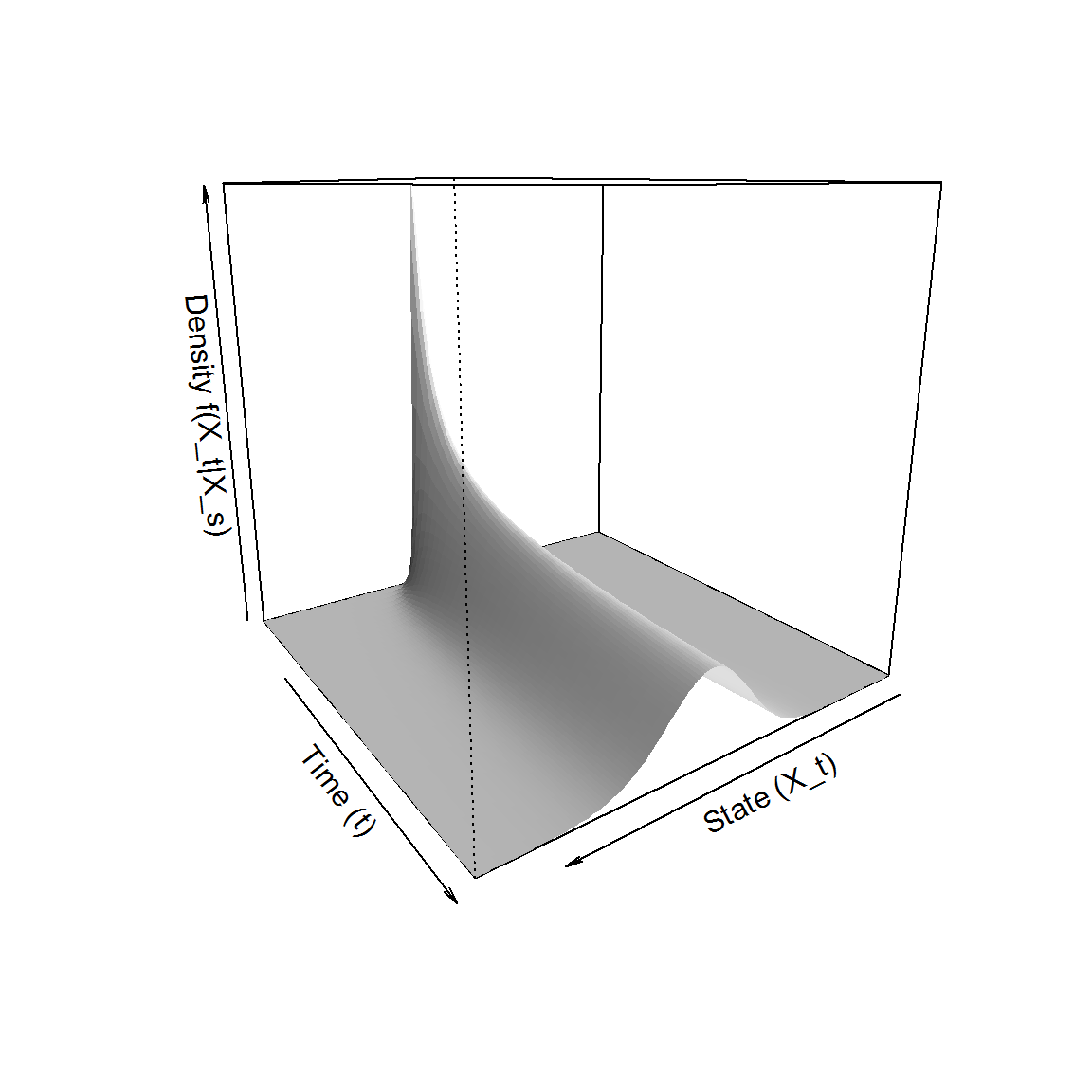Interestingly, the transitional density of the BAJD appears to be superficially very similar to that of a CIR process. However, as will be seen in the examples that follow, the presence of the jump mechanism significantly affects the nature of the transition density. ## Time-Inhomogeneous CIR with state-dependent jump intensity A common pitfall when modelling real-world phenomena with diffusion processes is the disparity between the assumptions under which a model is constructed and that which is observed in the data, for example the constant volatility assumption in the Black-Scholes methodology. However, such assumptions are often required to make a given theory mathematically tractable. In the context of jump diffusion models, it may for example be more realistic that the intensity at which jumps arrive in the data is related to the level of the process at any given time. For example, consider a jump diffusion model governed by the SDE: \begin{aligned} dX_t &= \kappa(\beta_0+\beta_1\sin(2\pi t)-X_t)dt +\sigma \sqrt{X_t}dB_t+dP_t\\ dP_t &= \dot{z}_tdN_t\\ \end{aligned} where the $$N_t$$ has state dependent intensity $$\lambda(X_t,t)= \lambda_0+\lambda_1X_t$$ and $$\dot{z}_t\sim \mbox{Nu}(\mu_z,\sigma_z^2)$$. Here we have assumed that the jump arrivals are linearly dependent on the state of the process. Within the GQD framework, we define the model in terms of its coefficient functions: library(DiffusionRjgqd) # Some parameter values: kap <- 1; beta_0 <- 5; beta_1 <- 3; sigma <- 0.15; lam_0 <- 0.5; lam_1 <- 0.1; mu_z <- 0.5; sigma_z <- 0.2; # Define the model: JGQD.remove() ##  "Removed : G0 G1 Q0 Q1 Lam0 Jmu Jsig Jlam" # Diffuse part G0 <- function(t){kap*(beta_0+beta_1*sin(2*pi*t))} G1 <- function(t){-kap} Q1 <- function(t){sigma^2} # Jump part Lam0 <- function(t){lam_0} Lam1 <- function(t){lam_1} Jmu <- function(t){mu_z} Jsig <- function(t){sigma_z} Note again the addition of the coefficient functions for the jump distribution Jmu(t) and Jsig(t), here reflecting the parameters of the Normal jump density (see the list of reserved function names for various jump distributions in the introductory vignette). Subsequently, by supplying initial values and the perimeters of the space on which we would like to evaluate the transitional density to the JGQD.density() function, we can calculate the transition density approximation.  Xt <- seq(2,9,1/20) Xs <- 6 t <- 5 s <- 0 dt <- 1/100 M <- JGQD.density(Xs,Xt,s,t,dt, Jdist = 'Normal') ## ## ================================================================ ## Jump Generalized Quadratic Diffusion (JGQD) ## ================================================================ ## _____________________ Drift Coefficients _______________________ ## G0 : kap*(beta_0+beta_1*sin(2*pi*t)) ## G1 : -kap ## G2 ## ___________________ Diffusion Coefficients _____________________ ## Q0 ## Q1 : sigma^2 ## Q2 ## _______________________ Jump Mechanism _________________________ ## ......................... Intensity ............................ ## Lam0 : lam_0 ## Lam1 : lam_1 ## Lam2 ## ........................... Jumps .............................. ## Normal ## Jmu : mu_z ## Jsig : sigma_z ## __________________ Distribution Approximant ____________________ ## Density approx. : Saddlepoint ## Trunc. Order : 8 ## Dens. Order : 4 ## =================================================================  persp(x=MXt,y=M$time,z=M$density,col='white',xlab='State (X_t)',ylab='Time (t)', zlab='Density f(X_t|X_s)',border=NA,shade=0.5,theta=145)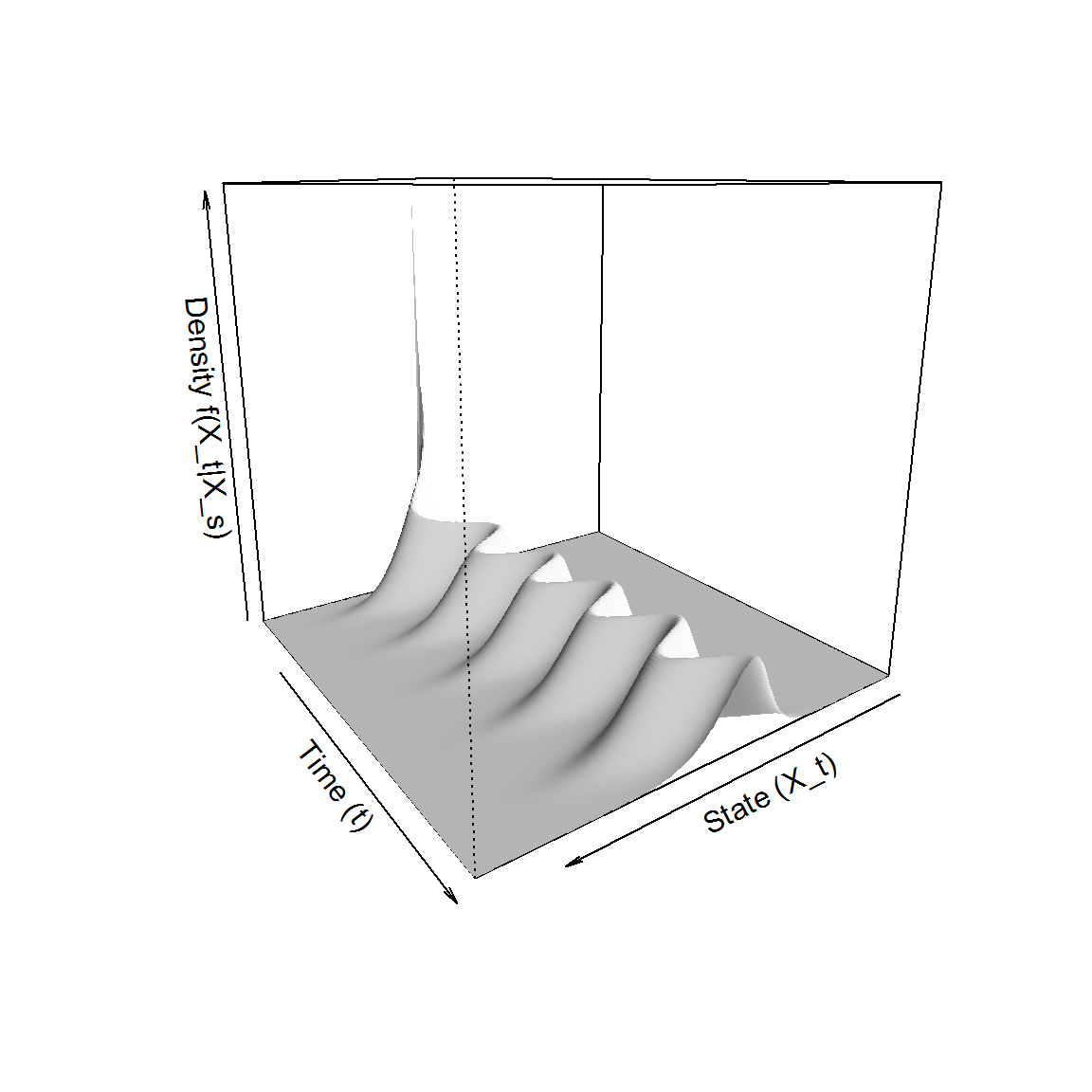Note that, since the transition horizon is large we have not specified that the excess factorization need be used. If we wish to invoke the excess factorization we can specify this through the factorize parameter:

 Xt <- seq(5.5,8,1/50)
t  <- 0.2
M2  <- JGQD.density(Xs,Xt,s,t,dt, Jdist = 'Normal',factorize = TRUE, print.output = FALSE)
## =================================================================
 # Plot the transitional density at t =0.2 and the evolution of the zero
# jump probability
par(mfrow=c(1,2))
plot(M2$density[,20]~M2$Xt,type='l',main ='Transition density at t = 0.2')
plot(M$zero_jump_prob~M$time,type='l',main =expression(P(N[t]-N[s]==0)))

# Superimpose the short horizon on the probability trajectory:
abline(v=t,lty='dotted')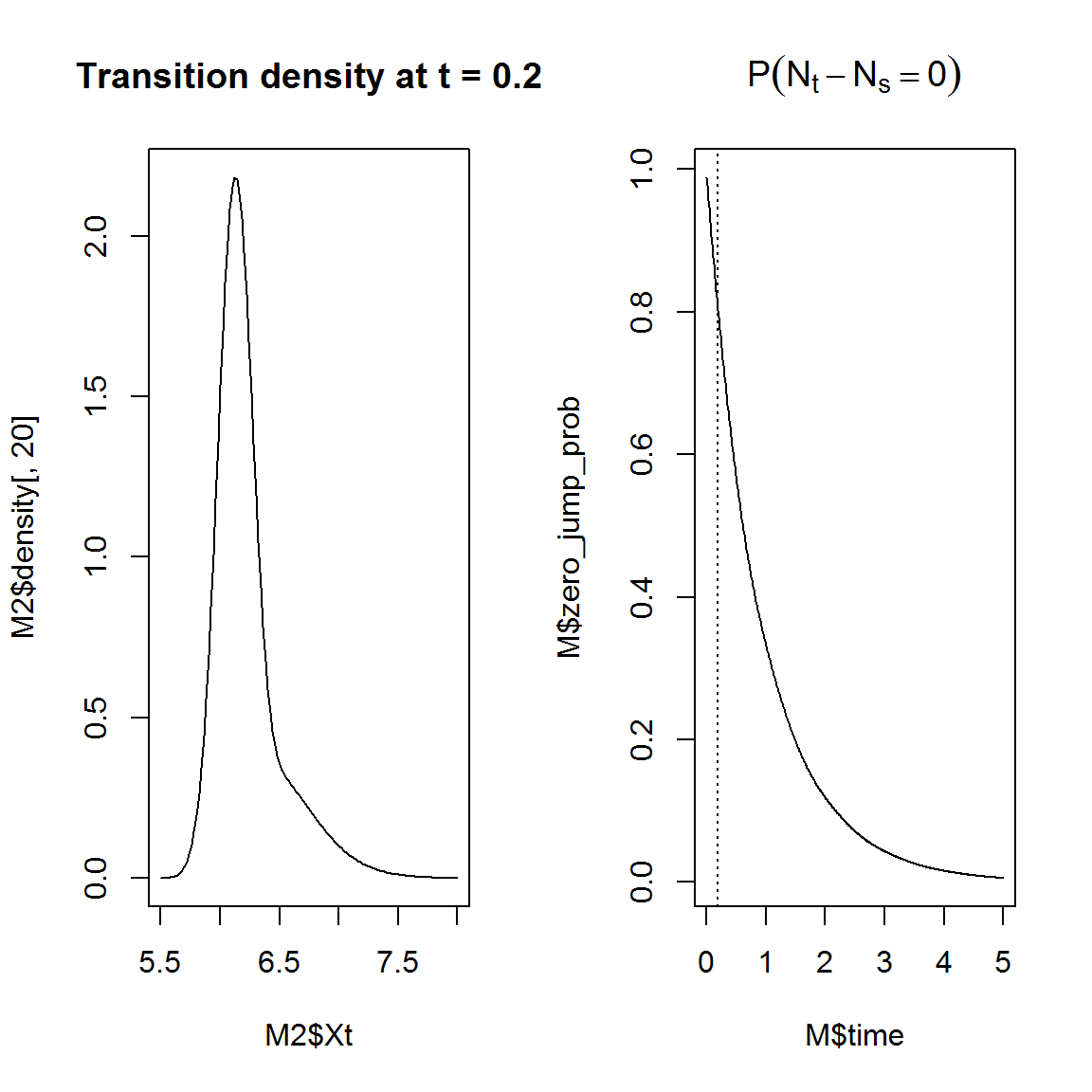Note the skew shape of the transitional density due to the presence of the jump mechanism. On short transition horizons, the jump mechanism has a significant effect on the nature of the transitional density: More often than not the transitional density will be multimodal and/or exhibit heavy tails. As such it is imperative that the excess factorization be used when approximating the transitional density on short transition horizons using the moment truncation methodology. For example, compare the jump diffusion transitional density to that of its purely diffuse counterpart:

 # Package for purely diffuse GQDs:
library(DiffusionRgqd)

M3  <- GQD.density(Xs,Xt,s,t,dt, print.output = FALSE)
## =================================================================
 plot(M2$density[,20]~M2$Xt,type='l',ylim=c(0,2.8),main ='Transition density at t = 0.2')
lines(M3$density[,20]~M3$Xt,col='blue',lty='dashed')
legend('topright',legend = c('Jump','Diffuse'),col=c(1,4),lty=c('solid','dashed'))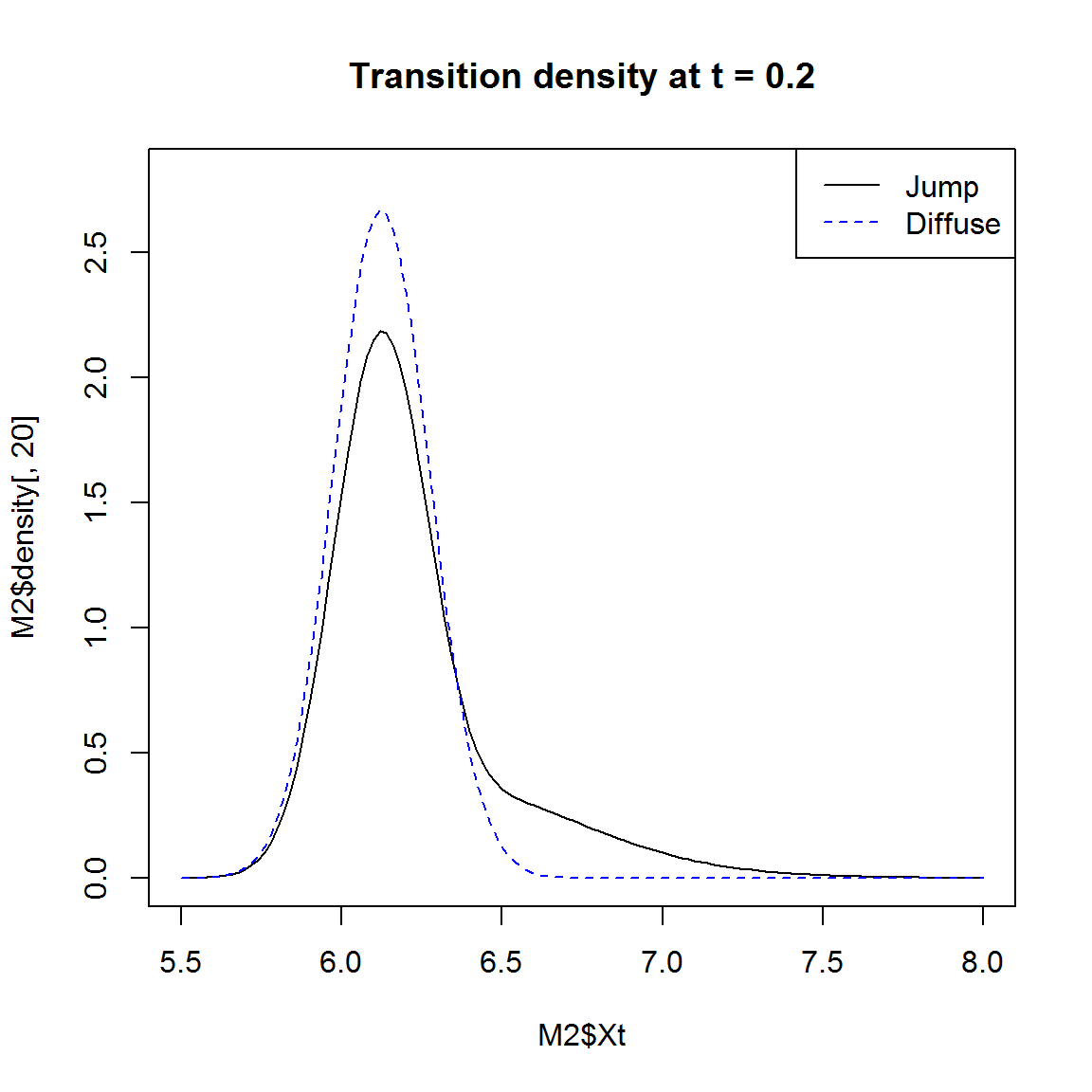## Stochastic jump intensity

Using the GQD framework, it is possible to construct complicated time inhomogeneous structures, even within the jump mechanism. For example, it is possible to construct a jump mechanism for which the intensity process is governed by some external stochastic process. For example, consider again a CIR process with additive jumps, but in this case let the intensity parameter be a two-state continuous time Markov-chain (CTMC). That is: \begin{aligned} dX_t &= \theta_1(\theta_2+\theta_3\sin(\pi t)-X_t)dt+\theta_4\sqrt{X_t}dB_t +dP_t\\ dP_t &= \dot{z}dN_t\\ \end{aligned} with $$\dot{z}\sim \mbox{N}(\theta_5,\theta_6^2)$$ and $$N_t \sim \mbox{PoiP}(\dot{y}_t)$$ where the intensity parameter $$\dot{y}_t$$ has dynamics given by a continuous time Markov-chain: $\dot{y}_t = \begin{cases} \lambda_1& \mbox{ State 1: Low jump frequency, }\\ \lambda_2& \mbox{ State 2: High jump frequency. }\\ \end{cases}$ with transition rate matrix: $R= \begin{pmatrix} -\beta_1 &\quad\beta_1\\ \quad\beta_2 &-\beta_2\\ \end{pmatrix}.$

For this example we let $$\boldsymbol\theta = \{2,5,2,1,1,0.25\}$$, $$\boldsymbol\lambda =\{\lambda_1,\lambda_2\} = \{1,3\}$$ and $$\boldsymbol\beta = \{\beta_1,\beta_2\} = \{0.25,1\}$$ and fix the initial values of the process to $$X_0 = 4$$ and $$\dot{y}_0 = \lambda_1$$. It is worth noting that the initial state of the intensity process will have an effect on the evolution of the transition density. For example, if the initial value of the intensity process was set to $$\lambda_2$$ the probability of a jump occurring would increase significantly over the transition horizon. Consequently, the transitional density, given that we start out in the high frequency jump regime is markedly different to the low-frequency regime. Furthermore, if the initial state for the intensity parameter $$\dot{y}_t$$ is not known, we may account for this latency by considering a mixture distribution for the transitional density: In this case one would construct the transition density by calculating the transitional density for both intensity regimes and subsequently weight each distribution according to the stationary distribution of the CTMC (or the appropriate initial distribution).

Since the intensity process now depends on an external process we need to modify the Kolmogorov forward eqn slightly: \begin{aligned} \frac{\partial}{\partial t} f(X_t|X_s) =& -\frac{\partial}{\partial X_t} [\mu(X_t,t) f(X_t|X_s)]+\frac{1}{2}\frac{\partial^2}{\partial X_t^2} [\sigma^2(X_t,t) f(X_t|X_s)]\\ &+\int\int\lambda(X_t-\dot{z}_t,\dot{y}_t)f(X_t-\dot{z}_t|X_s)d\phi(\dot{z}_t)d\pi(\dot{y}_t)d\dot{z}_td\dot{y}_t-\int\int\lambda(X_t,\dot{y}_t)f(X_t|X_s)d\phi(\dot{z}_t)d\pi(\dot{y}_t)d\dot{z}_td\dot{y}_t. \end{aligned}

From this, it can be shown that in order to evaluate the moment equations for this process, we require the evolution of the expectation of the intensity process over time. For the present example an analytical expression for the expectation of the intensity parameter assumes the form: $E(\dot{y}_t) = \begin{cases} \lambda_1\frac{\beta_2+\beta_1e^{-(\beta_1+\beta_2)t}}{\beta_1+\beta_2}+\lambda_2\frac{\beta_1(1-e^{-(\beta_1+\beta_2)t})}{\beta_1+\beta_2}& \mbox{ if } \dot{y}_0 = \lambda_1,\\ \lambda_2\frac{\beta_1+\beta_2e^{-(\beta_1+\beta_2)t}}{\beta_1+\beta_2}+\lambda_1\frac{\beta_2(1-e^{-(\beta_1+\beta_2)t})}{\beta_1+\beta_2}& \mbox{ if } \dot{y}_0 = \lambda_2.\\ \end{cases}$ Thus, in R:

JGQD.remove()
##  "Removed :  G0 G1 Q1 Lam0 Lam1 Jmu Jsig"
G0=function(t){2*5+2*sin(1*pi*t)}
G1=function(t){-2}
Q1=function(t){1}

l1 <- 1
l2 <- 3
rho1 <- 0.25
rho2 <- 1

Jmu  <- function(t){1}
Jsig <- function(t){0.25}
Lam0 <- function(t){l1*(rho2+rho1*exp(-(rho1+rho2)*t))/(rho1+rho2)+l2*rho1/(rho1+rho2)*(1-exp(-(rho1+rho2)*t))}
t <- seq(0,5,1/100)
# Intensity assumes the expectation trajectory of the intensity process:
plot(Lam0(t)~t,type='l')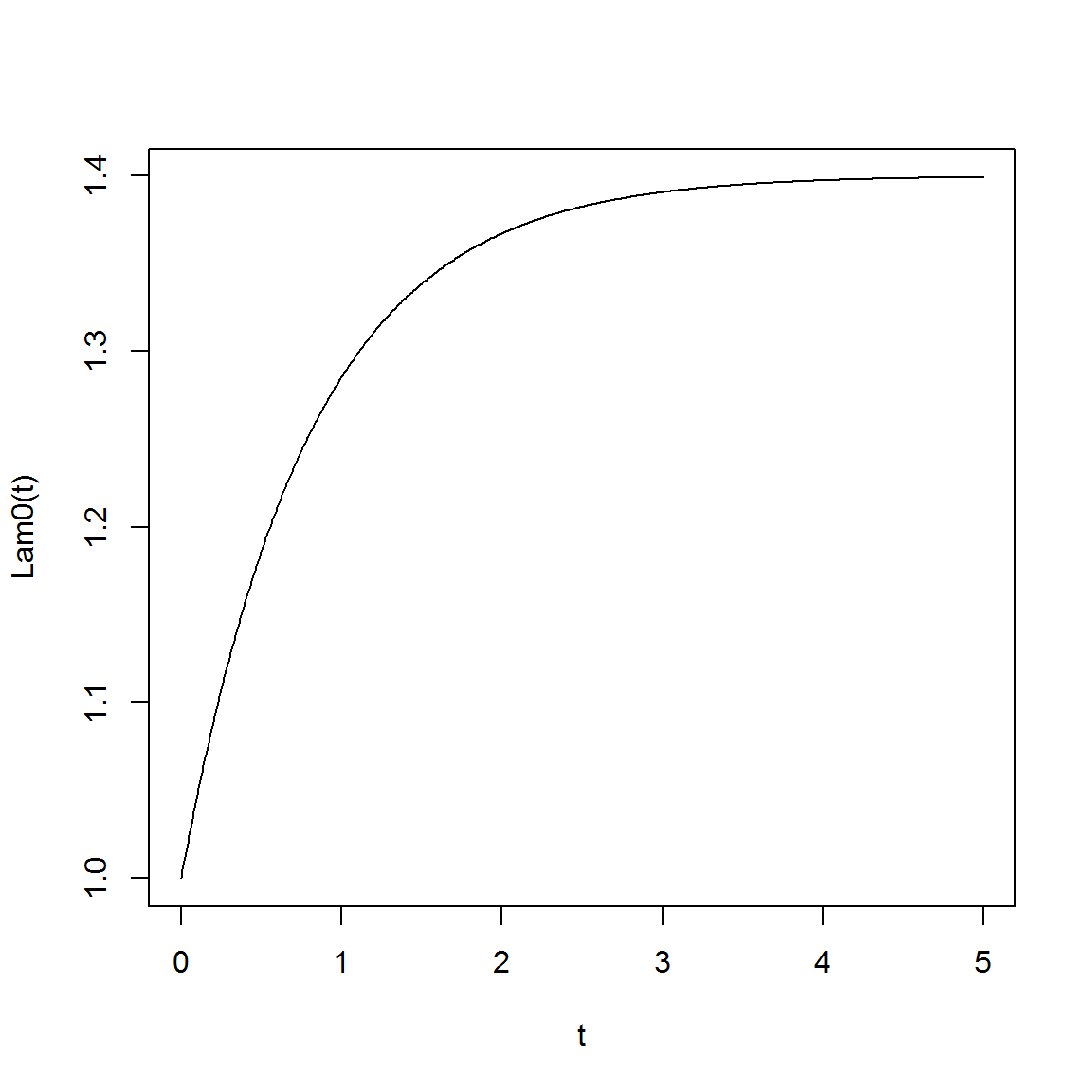TT  <- 5
res <- JGQD.density(4,seq(2,14,1/10),0,TT,1/100)
##
##  ================================================================
##             Jump Generalized Quadratic Diffusion (JGQD)
##  ================================================================
##  _____________________ Drift Coefficients _______________________
##  G0 : 2*5+2*sin(1*pi*t)
##  G1 : -2
##  G2
##  ___________________ Diffusion Coefficients _____________________
##  Q0
##  Q1 : 1
##  Q2
##  _______________________ Jump Mechanism _________________________
##  ......................... Intensity ............................
##  Lam0 : l1*(rho2+rho1*exp(-(rho1+rho2)*t))/(rho1+rho2)+l2*rho1/(rho1+rho2)*(1-exp(-(rho1+rho2)*t))
##  Lam1
##  Lam2
##  ........................... Jumps ..............................
##  Normal
##  Jmu : 1
##  Jsig : 0.25
##  __________________ Distribution Approximant ____________________
##  Trunc. Order    : 8
##  Dens.  Order    : 4
## =================================================================

For comparative purposes we may compare simulated trajectories of the moments of the process and compare the simulated trajectory of the transitional density to that of the approximation. This can be achieved using a (somewhat oversimplified) simulation algorithm:

#' Now simulate the jump diffusion

mu     <- function(x,t){G0(t)+G1(t)*x} # Drift
sigma  <- function(x,t){sqrt(Q1(t)*x)} # Diffusion
j      <- function(x,z){z}             # Jumps
simulate <- function(x0=4,N=25000,pts = 1:4)
{
d=0
delta=1/1000
tt=seq(0,TT,delta)
X=rep(x0,N)

kkk=1
MM= matrix(0,4,length(tt))
MM[,1]=X^{1:4}

xtrak = rep(x0,length(tt))
jtrak = rep(0,length(tt))
etrak = rep(0,length(tt))
ltrak = rep(0,length(tt))
sttes = rep(l1,N)
L=list()
kkk =1
p.states = jtrak
e.states = jtrak
for(i in 2:length(tt))
{
X=X+mu(X,d)*delta+sigma(X,d)*rnorm(N,sd=sqrt(delta))
d=d+delta

events = ((sttes)*delta*exp(-(sttes)*delta)>runif(N))
xpre=X
if(any(events))
{
wh=which(events)
X[wh]=X[wh]+j(X[wh],rnorm(length(wh),Jmu(d),Jsig(d)))

}
jtrak[i] = X-xpre
xtrak[i]  = X

prbs1=rho1/(rho1+rho2)*(1-exp(-(rho1+rho2)*delta))
prbs2=rho2/(rho1+rho2)*(1-exp(-(rho1+rho2)*delta))

whh1=which(sttes==l1)
whh2=which(sttes==l2)
if(length(whh1)!=0)
{
whh1.2=which(runif(length(whh1))<prbs1)
if(length(whh1.2)!=0)
{
sttes[whh1][whh1.2]=l2
}
}
if(length(whh2)!=0)
{
whh2.2=which(runif(length(whh2))<prbs2)
if(length(whh2.2)!=0)
{
sttes[whh2][whh2.2]=l1
}
}

p.states[i] = mean(sttes==l2)
e.states[i] = mean(sttes)
etrak[i]   = sttes

MM[1,i]=sum(X)/N
MM[2,i]=sum(X^2)/N
MM[3,i]=sum(X^3)/N
MM[4,i]=sum(X^4)/N
if(sum(round(pts,3)==round(d,3))!=0)
{
L[[kkk]] = hist(X,plot=F,breaks=25)
kkk=kkk+1
}
}
return(list(MM=MM,tt=tt,X=X,xtrak=xtrak,etrak=etrak,jtrak=jtrak,ltrak=ltrak,hists=L,p.states=p.states,e.states=e.states))
}
res2 <- simulate()

Subsequently, we can have a look at what a typical trajectory for such a process looks like, and compare the moment trajectories of the simulated process and those of the moment truncation method:

 par(mfrow=c(3,1))
plot(res2$xtrak~res2$tt,type='l',col='blue',main='Trajectory',xlab = 'time',ylab ='X_t')
plot(res2$jtrak~res2$tt,type='h',col='black',ylim=c(0,3),lwd=2,main='Jumps',xlab ='time',ylab ='Z_t')
plot(res2$etrak~res2$tt,type='s',col='black',ylim=c(0,6),lwd=1,main='Intensities',xlab ='time',ylab ='Z_t')
abline(h =c(l1,l2),lty='dotted',col='lightgrey')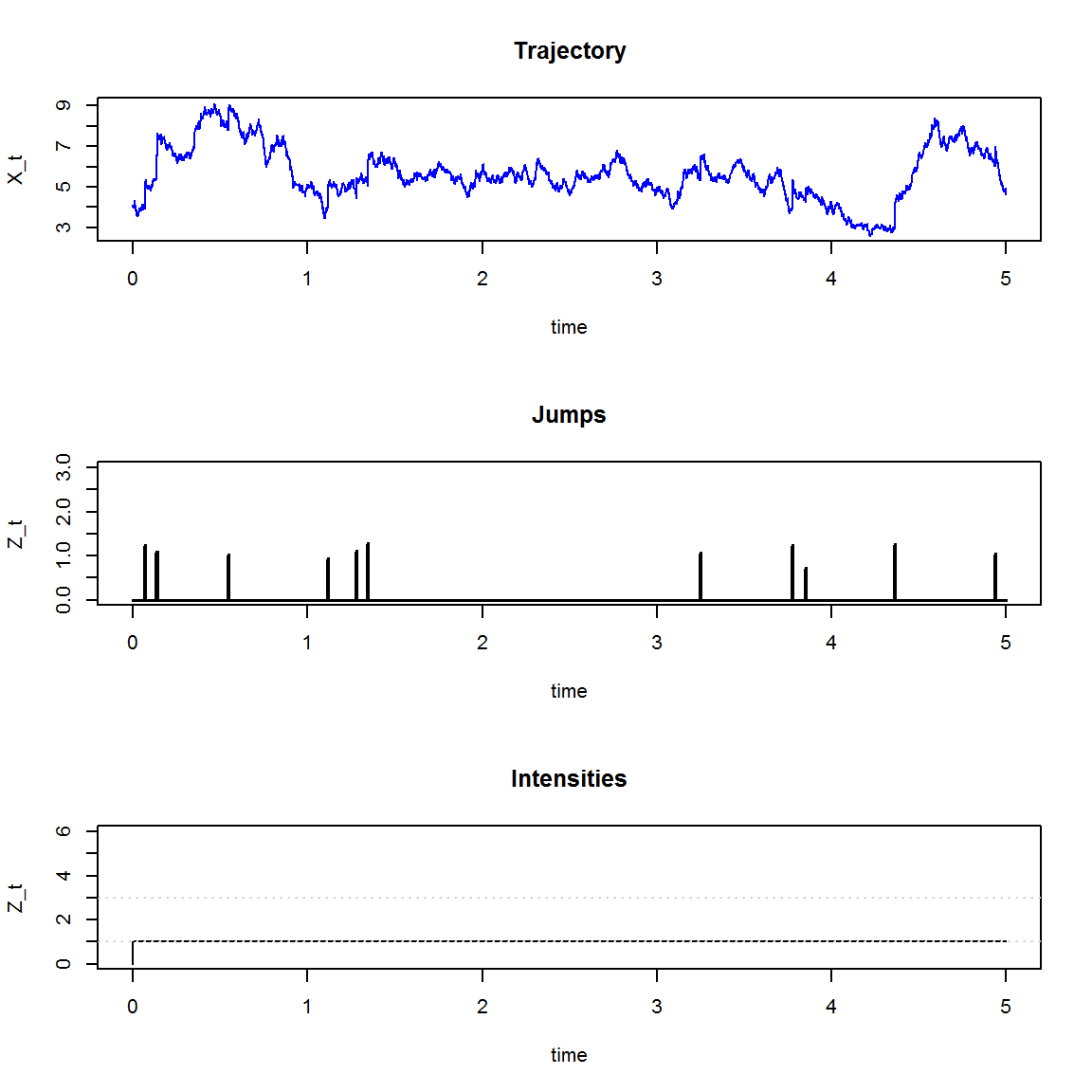par(mfrow=c(2,2))
for(i in 1:4)
{
plot(res2$MM[i,]~res2$tt,type='l',main='Moment trajectory',xlab='Time (t)',
ylab=paste0('m_',i,'(t)'))
lines(res$moments[i,]~res$time,lty='dashed',col='blue',lwd=2)
}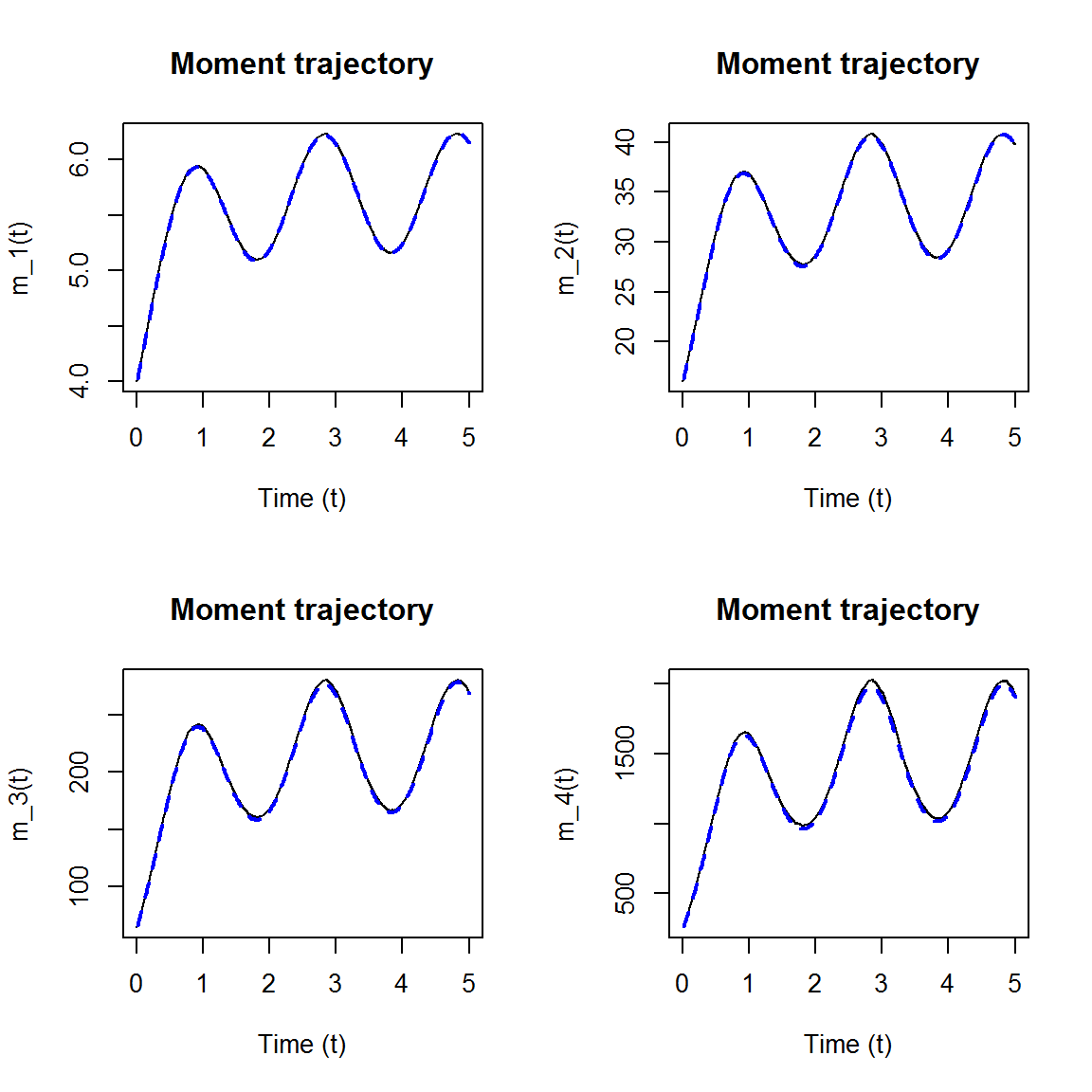Finally we can compare the simulated transitional density to that of the simulated process:

 persp(x=res$Xt,y=res$time,z=res$density,col='white',xlab='State (X_t)',ylab='Time (t)', zlab='Density f(X_t|X_s)',border=NA,shade=0.5,theta=145)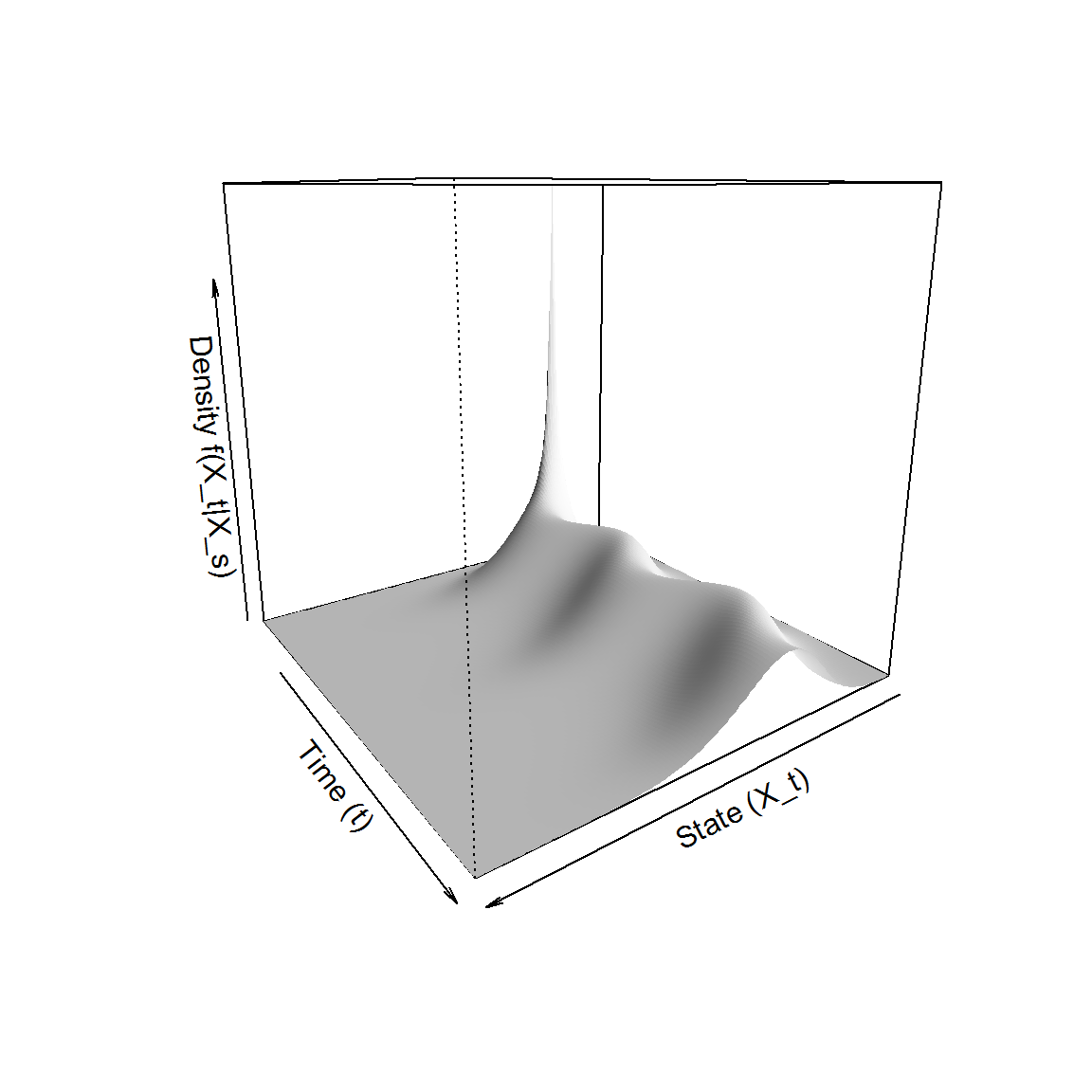par(mfrow=c(2,2)) for(i in 1:4) { plot(res2$hists[[i]]$density~c(res2$hists[[i]]$mids-diff(res2$hists[[i]]$mids) / 2), type = 's',lty = 'solid', lwd = 1, xlab = 'time', ylab = 'Density', main = paste('Density at time t =',i)) lines(res$density[,i*100]~res\$Xt,col='darkblue')
}